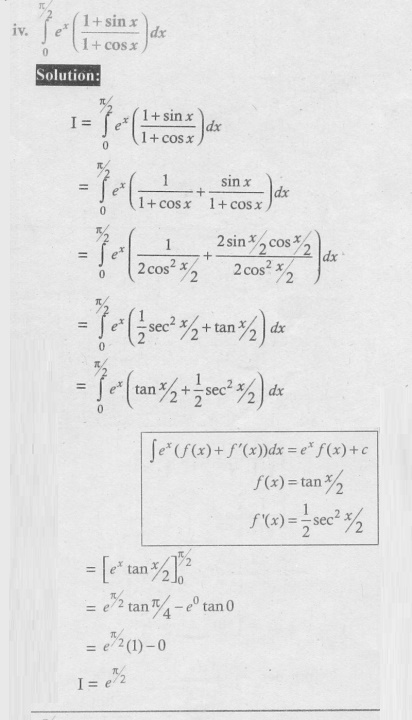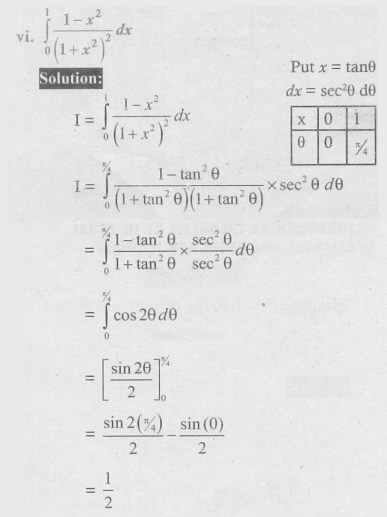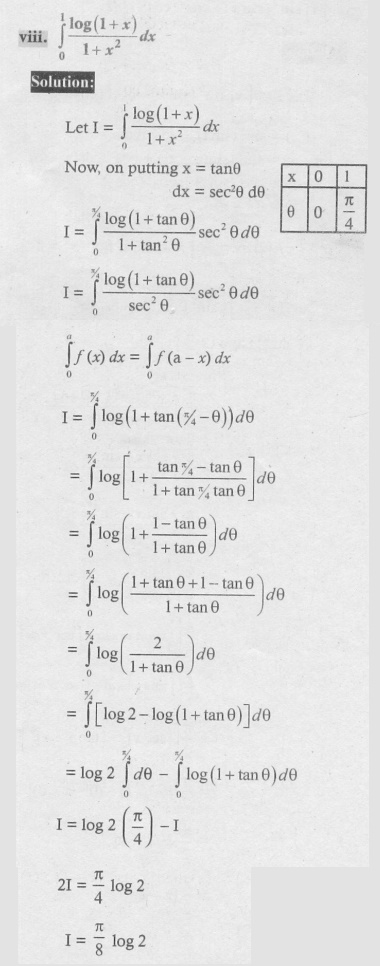Home | | Maths 12th Std | Exercise 9.3: Fundamental Theorems of Integral Calculus and their Applications

# Exercise 9.3: Fundamental Theorems of Integral Calculus and their Applications

Maths Book back answers and solution for Exercise questions - Mathematics : Applications of Integration : Fundamental Theorems of Integral Calculus and their Applications - Exercise Problem Questions with Answer, Solution

EXERCISE 9.3

1. Evaluate the following integrals using properties of integration :2. Evaluate the following integrals using properties of integration :